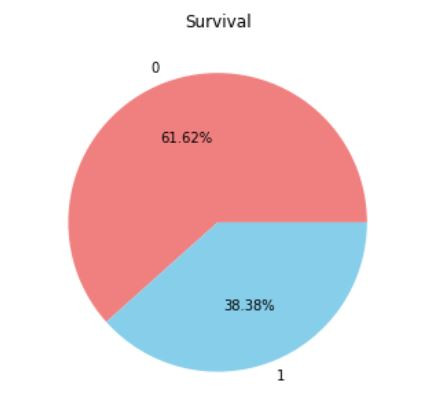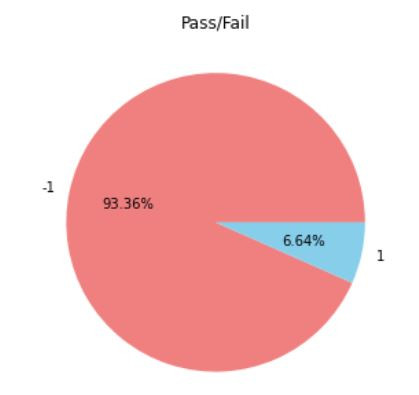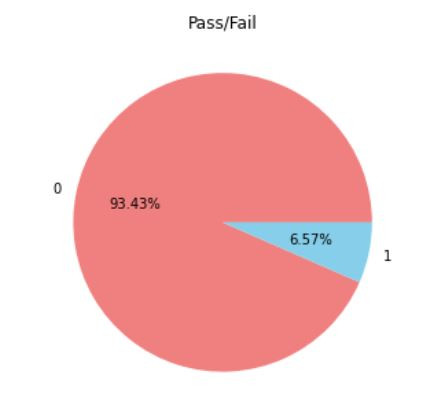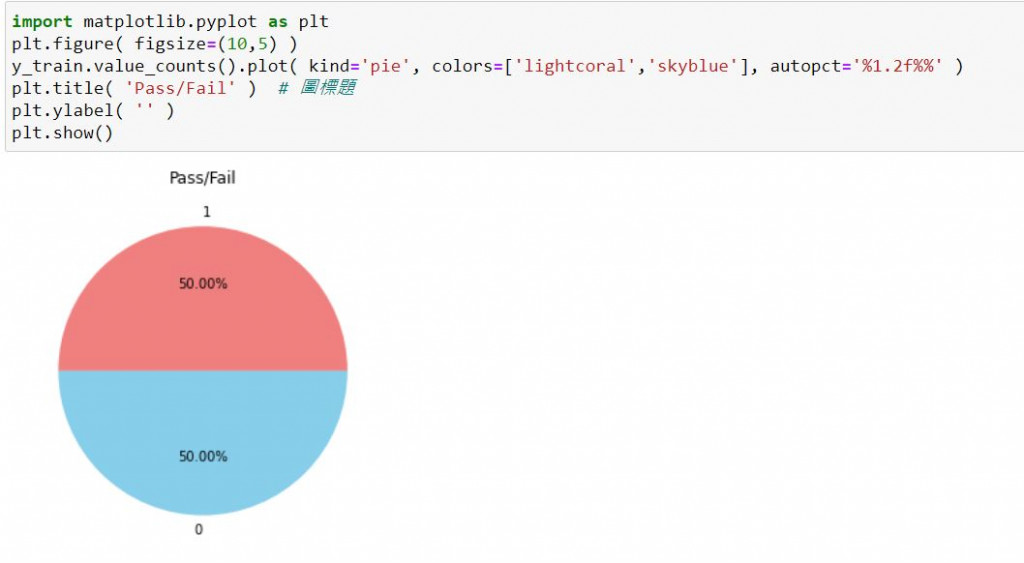DAY 11
1
AI & Data

一、不平衡資料(Imbalanced Data)

利用套件看出特徵的資料量

train_df['Survived'].value_counts()畫出圓餅圖查看樣本比例

import matplotlib.pyplot as plt
plt.figure( figsize=(10,5) )
train_df['Survived'].value_counts().plot( kind='pie', colors=['lightcoral','skyblue'], autopct='%1.2f%%' )
plt.title( 'Survival' )  # 圖標題
plt.ylabel( '' )
plt.show()這是一份半導體製造的良率分析，-1代表Pass，1代表Fail，資料占比如下三、上採樣(OverSampling)

先分出訓練集及測試集

import numpy as np
from sklearn.model_selection import train_test_split
X_train, X_test, y_train, y_test = train_test_split(x, y, test_size=0.3,random_state=11)

接著看一下資料的占比

import matplotlib.pyplot as plt
plt.figure( figsize=(10,5) )
y_train.value_counts().plot( kind='pie', colors=['lightcoral','skyblue'], autopct='%1.2f%%' )
plt.title( 'Pass/Fail' )  # 圖標題
plt.ylabel( '' )
plt.show()做SMOTE處理

from imblearn.over_sampling import SMOTE
X_train, y_train = SMOTE().fit_resample(X_train, y_train)

看看成果